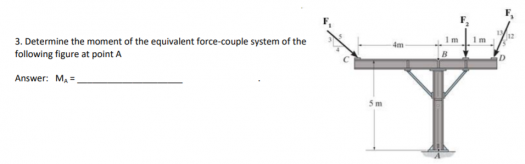# How to find the values of the following forces when it isn't given?

2 posts / 0 new
Alun
How to find the values of the following forces when it isn't given?

How to find the values of the following forces when it isn't given?Jhun VertI think you just express the moment at A in terms of Fn.

Assuming counterclockwise moment as positive:
$M_A = 4\left( \frac{3}{5}F_1 \right) - 5\left( \frac{4}{5}F_1 \right) - 1(F_2) + 5\left( \frac{5}{13}F_3 \right) + 2\left( \frac{12}{13}F_3 \right)$

• Mathematics inside the configured delimiters is rendered by MathJax. The default math delimiters are $$...$$ and $...$ for displayed mathematics, and $...$ and $...$ for in-line mathematics.